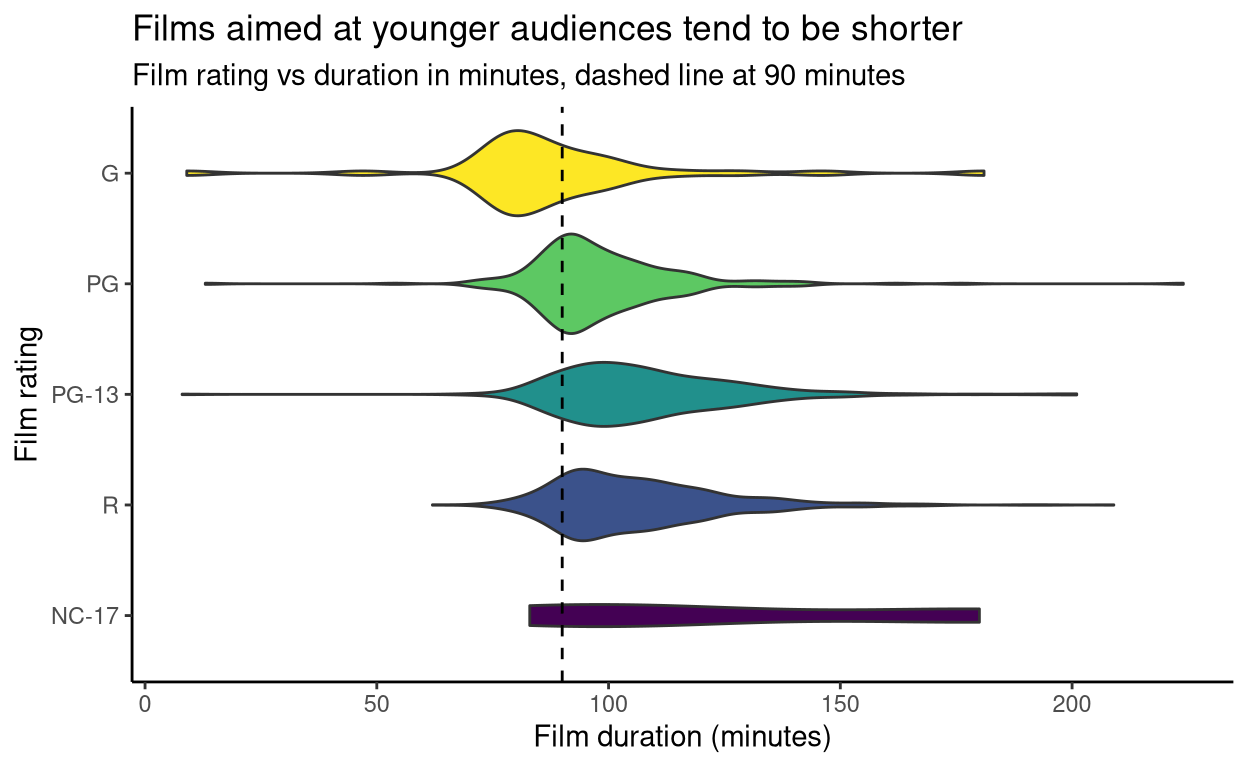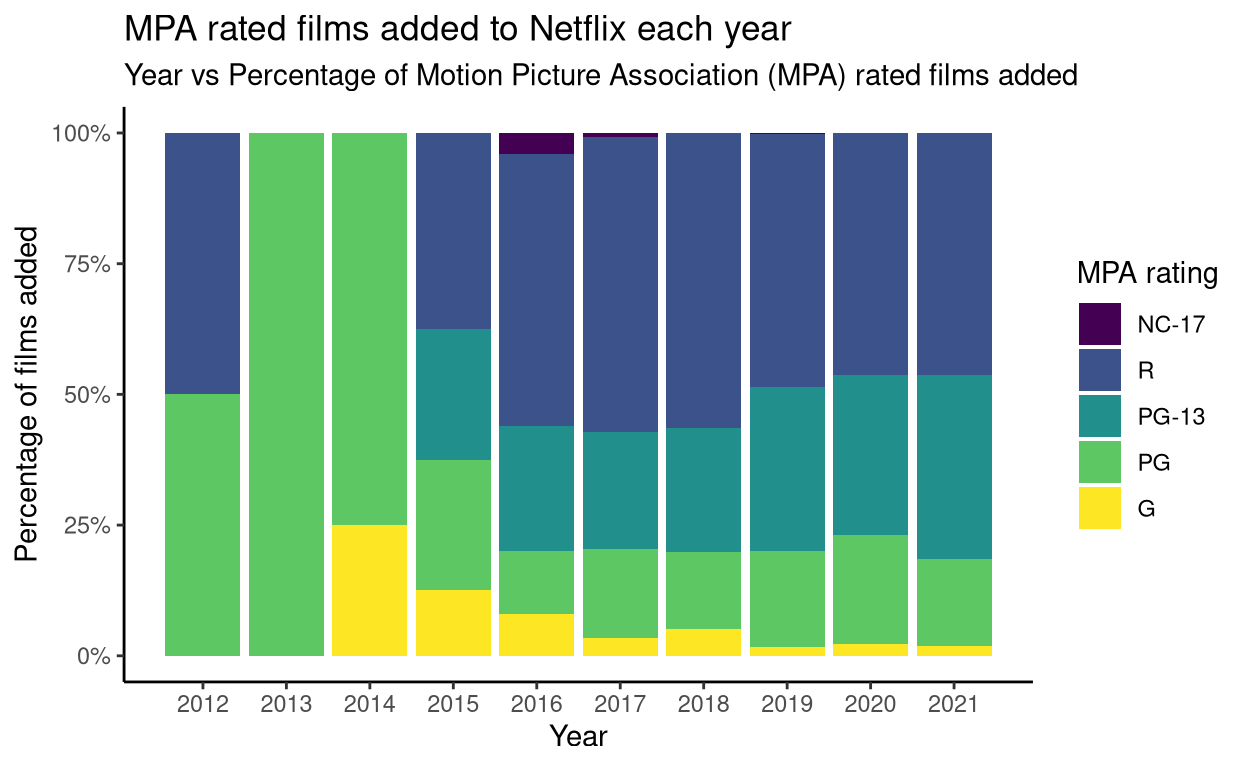Want to share your content on R-bloggers? click here if you have a blog, or here if you don't.

## Setup

Loading the `R` libraries and data set.

```# Loading libraries
library(tidyverse)
library(tidytext)
library(tidytuesdayR)
library(forcats)

Wrangling data for visualisation.

```# Selecting the films in the data set, creating a numeric variable for film
## duration called "runtime", creating a numeric variable for year added
netflix_movies <- tt\$netflix_titles %>%
filter(type == "Movie") %>%
mutate(runtime = as.numeric(str_sub(duration, end = -5))) %>%

# Creating a vector of MPA film ratings
MPA_ratings <- c("G", "PG", "PG-13", "R", "NC-17")

# Creating a list of the top categories ("listed in") on Netflix
top_listings <- tt\$netflix_titles %>%
separate_rows(listed_in, sep = ", ") %>%
count(listed_in, sort = TRUE) %>%
select(listed_in) %>%
# Counting the occurrences of words in the descriptions of these top categories
top_listing_words <- tt\$netflix_titles %>%
separate_rows(listed_in, sep = ", ") %>%
filter(listed_in %in% top_listings\$listed_in) %>%
select(listed_in, description) %>%
unnest_tokens(word, description) %>%
anti_join(stop_words, by = "word") %>%
count(listed_in, word, sort = TRUE)
# Counting the total number of words in the descriptions of these top categories
total_words <- top_listing_words %>%
group_by(listed_in) %>%
summarise(total = sum(n))
# Adding word totals to individual word counts
top_listing_words <- left_join(top_listing_words, total_words, by = "listed_in")
# Adding tf-idf to these word counts
top_listing_words <- top_listing_words %>%
bind_tf_idf(word, listed_in, n)```

## Films for younger audiences tend to be shorter

For this plot, I selected the films in the data set which have Motion Picture Association (MPA) ratings. This is the American film rating system, with categories as follows:

• G General Audiences
• PG Parental Guidance Suggested
• PG-13 Parents Strongly Cautioned
• R Restricted

When the duration of these films are plotted according to their MPA rating, we can see that films aimed at older audiences tend to be longer than those aimed at younger viewers.

```# Plotting distributions of film length according to MPA rating
netflix_movies %>%
filter(type == "Movie" & !is.na(rating)) %>%
filter(rating %in% MPA_ratings) %>%
mutate(rating = factor(rating, levels = rev(MPA_ratings))) %>%
ggplot(aes(x = rating, y = runtime, fill = rating)) +
geom_violin() +
geom_hline(yintercept = 90, linetype = 2) +
coord_flip() +
theme_classic() +
scale_fill_viridis_d() +
theme(legend.position = "none") +
labs(x = "Film rating", y = "Film duration (minutes)",
title = "Films aimed at younger audiences tend to be shorter",
subtitle = "Film rating vs duration in minutes, dashed line at 90 minutes")```## Netflix is adding fewer films for kids

For this plot, I selected MPA rated films again, and the year they were added to Netflix. Each column represents a single year, and each column is divided according to the percentage of films in each MPA rating added that year. From this plot, we can see that Netflix is adding fewer films for kids (G and PG).

```# Summarising films added to Netflix annually by MPA rating
netflix_movies %>%
filter(rating %in% MPA_ratings & !is.na(year_added)) %>%
count(rating) %>%
mutate(percentage = n / sum(n)) %>%
mutate(rating = factor(rating, levels = rev(MPA_ratings))) %>%
ggplot(aes(x = year_added, y = percentage, fill = rating)) +
geom_col() +
theme_classic() +
scale_fill_viridis_d() +
scale_x_continuous(breaks = seq(2008, 2021, by = 1)) +
scale_y_continuous(labels = scales::percent_format(scale = 100)) +
labs(y = "Percentage of films added", x = "Year", fill = "MPA rating",
title = "MPA rated films added to Netflix each year",
subtitle = "Year vs Percentage of Motion Picture Association (MPA) rated films added")```## Significant words in category descriptions

In this section, significant words used to describe titles in the most common Netflix categories are plotted. This is done by…

• taking the descriptions of films/shows listed in these common categories as a corpus
• splitting that corpus into a document for each category
• calculating tf-idf to find significant words used to describe titles in each category

From this plot, we can see that documentaries are associated with the most unique words. “Action & Adventure” titles are associated with “guts”, “revenge” and “007”. “International TV Shows” are associated with “Spain’s”, “parallel” and “docuseries”.

This plot is confounded by Netflix titles having multiple categories. For example, a crime show may have “Crime TV Shows” and “TV Dramas” as its categories. This interferes with detecting significant words used in descriptions according to category. In this plot, “documentary” is the most significant word used in the “Documentary” category, but it is also a significant word in the “International Movies” category.

```# Plotting significant words used in Netflix descriptions
top_listing_words %>%
slice_max(tf_idf, n = 20) %>%
ggplot(aes(tf_idf, fct_reorder(word, tf_idf), fill = listed_in)) +
geom_col() +
theme_classic() +
labs(y = "Word", x = "Term frequency-inverse document frequency (tf-idf)",
fill = "Netflix categories",
title = "Significant words in common Netflix category descriptions",
subtitle = "Words that appear often in these categories and not others")```